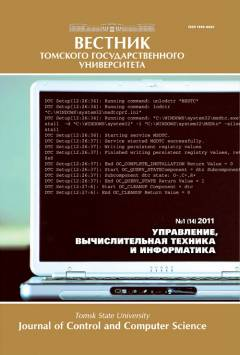# Вычислительные аспекты вероятностных расширений

В статье предлагается новый подход к вычислению функций со случайными аргументами. Подход основан на идее уменьшения размерности функции путем вычисления определенных интегралов и использования вычислительного вероятностного анализа. Применяется одно из основных понятий вычислительного вероятностного анализа - вероятностное расширение для вычисления функции со случайными аргументами. Для реализации этого метода предлагается способ, основанный на параллельных рекурсивных вычислениях. Приведены численные примеры, демонстрирующие эффективность предложенного подхода.

## Ключевые слова

computational probabilistic analysis, probabilistic extensions, non-Monte Carlo methods, random boundary value problem, вычислительный вероятностный анализ, вероятностные расширения, не Монте-Карло методы, случайные краевые задачи

## Авторы

 ФИО Организация Дополнительно E-mail Добронец Борис Станиславович Сибирский федеральный университет профессор, доктор физико-математических наук, профессор кафедры систем искусственного интеллекта BDobronets@yandex.ru Попова Ольга Аркадьевна Сибирский федеральный университет кандидат технических наук, доцент кафедры систем искусственного интеллекта OlgaArc@yandex.ru
Всего: 2

## Ссылки

Rocquigny, E. (2012) Modelling Under Risk and Uncertainty: An Introduction to Statistical, Phenomenological and Computational Methods. Wiley Series in Probability and Statistics. John Wiley & Sons.
Springer, M.D. (1979) The Algebra of Random Variables. New York; Chichester; Brisbane: John Wiley & Sons.
Gerasimov, V.A., Dobronets, B.S. & Shustrov M.Yu. (1991) Numerical operations of histogram arithmetic and their applications. Automation and Remote Control. 52(2). pp. 208-212.
Williamson, R. & Downs, T. (1990) Probabilistic arithmetic i: numerical methods for calculating convolutions and dependency bounds. International Journal of Approximate Reasoning. 4(2). pp. 89-158. DOI: 10.1016/0888-613X(90)90022-T
Li, W. & Hym, J. (2004) Computer arithmetic for probability distribution variables. Reliability Engineering and System Safety. 85(1-3). pp. 191-209. DOI: 10.1016/j.ress.2004.03.012
Jaroszewicz, S. & Korzen, M. (2012) Arithmetic operations on independent random variables: a numerical approach. SIAM J. Sci. Comput. 34(3). pp. A1241-A1265. DOI: 10.1137/110839680
Dobronets, B.S. & Popova, O.A. (2014) Numerical probabilistic analysis under aleatory and epistemic uncertainty. Reliable Computing. 19. pp. 274-289.
Dobronets, B. & Popova, O. (2016) Numerical Probabilistic Approach for Optimization Problems. Scientific Computing, Computer Arithmetic, and Validated Numerics. Lecture Notes in Computer Science. Vol. 9553. Springer International Publishing, Cham. pp. 43-53.
Dobronets, B.S. & Popova, O.A. (2017) Improving the accuracy of the probability density function estimation. Journal of Siberian Federal University, Mathematics and Physics. 10(1). pp. 16-21. DOI: 10.17516/1997-1397-2017-10-1-16-21
Dobronets, B.S. & Popova, O.A. (2018) Piecewise Polynomial Aggregation as Preprocessing for Data Numerical Modeling. IOP Conf. Series: Journal of Physics: Conf. Series. 1015. DOI:10.1088/1742-6596/1015/3/032028
Dobronets, B.S. & Popova, O.A. (2018) Improving reliability of aggregation, numerical simulation and analysis of complex systems by empirical data. IOP Conf. Series: Materials Science and Engineering. 354. D0I:10.1088/1757-899X/354/1/012006
Popova, O.A. (2019) Using Richardson extrapolation to improve the accuracy of procesing and analyzing empirical data. Measurement Techniques. 2. pp. 18-22.
Mikhailov, G.A. & Voitishek, A.V. (2018) Statisticheskoe modelirovanie: metodMonte-Karlo [Statistical modeling. Monte Carlo methods]. Moscow: Yurait.
Soong, T.T. (1973) Random Differential Equations in Science and Engineering. New York and London: Academic Press.